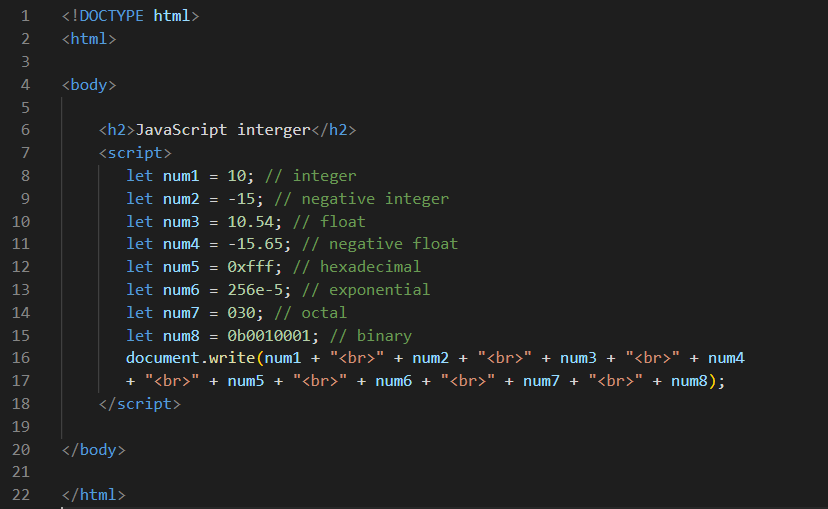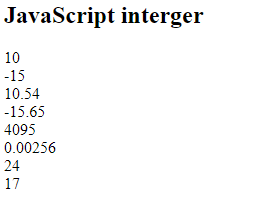JavaScript Integer | JavaScript Random Integer Tutorial By WDH

# JavaScript Integer

JavaScript Integer
Integers values that can be processed and calculated-either positive or negative, and floating point. Integers value will be accurate up to 15 digits in JavaScript. Integers with 16 digits onwards will be changed and rounded up or down; therefore, use BigInt for integers larger than 15 digits.
ExampleOutput